### Master Math with 1:1 Online Classes. Learn from Experts!# Multiplication Tables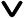• What are Times Tables?
• Multiplication Tables from 1 to 20
• Math Tables 1 to 5
• Times Tables 6 to 10
• Times Tables 11 to 15
• Times Tablets 16 to 20
• Multiplication chart
• How to read a Multiplication Chart?
• Why should you learn times tables?
• Easy tricks and tips to learn multiplication tables
• Printable Multiplication Chart
• Printable Multiplication Tables 1 to 20
• Solved Examples
• Practice Problems

Multiplication tables, also known as Times Tables or Math Tables, are a fundamental aspect of arithmetic. They consist of organized grids that showcase the results of multiplying numbers from 1 to 10 (or even higher) with each other. Learning these tables is a crucial step in mastering multiplication. They provide a quick reference for finding the product of two numbers and are essential for various mathematical calculations, from basic arithmetic to more advanced math. Mastery of multiplication tables is a foundational skill that greatly contributes to a student's math proficiency, fostering quick mental math and problem-solving abilities.

## What are Times Tables?

A times table or multiplication table is a list of multiples of a given number arranged in an ascending order. This list consists of multiplication sentences of a particular number multiplied with the numbers from 1 to 10, essentially representing the first ten multiples of that number.

## Multiplication Tables from 1 to 20

Multiplication tables form the foundation for various mathematical concepts and problem-solving skills. While most of us are familiar with the multiplication tables from 1 to 10, extending these tables to include numbers up to 20 opens up a world of mathematical possibilities. These expanded multiplication tables not only help students grasp the relationships between numbers but also serve as valuable tools for everyday calculations, from shopping and budgeting to more complex mathematical operations.

### Math Tables 1 to 5

Times tables from 1 to 5 are basic arithmetic skills and mastering times tables from 1 to 5 is like constructing a sturdy mathematical foundation upon which you can build your mathematical knowledge.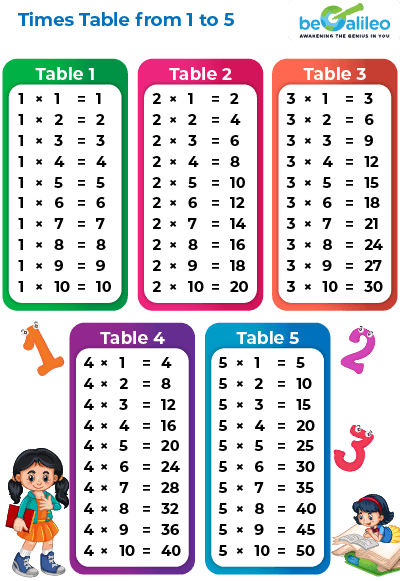### Times Tables 6 to 10

Multiplication tables from 6 to 10 mark an exciting progression in mathematical learning. As students advance through their mathematical journey, these tables become increasingly important.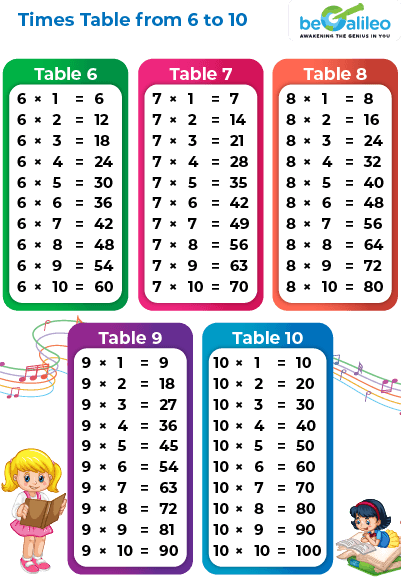### Times Tables 11 to 15

Multiplication tables from 11 to 15 introduce students to a higher level of multiplication complexity and open the door to more advanced mathematical concepts.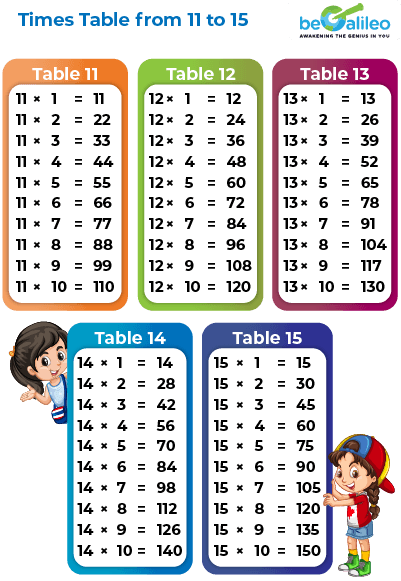### Times Tables 16 to 20

Multiplication tables from 16 to 20 represent the culmination of the foundational multiplication skill. They may initially seem more challenging but they offer valuable insights into the world of numbers and mathematics.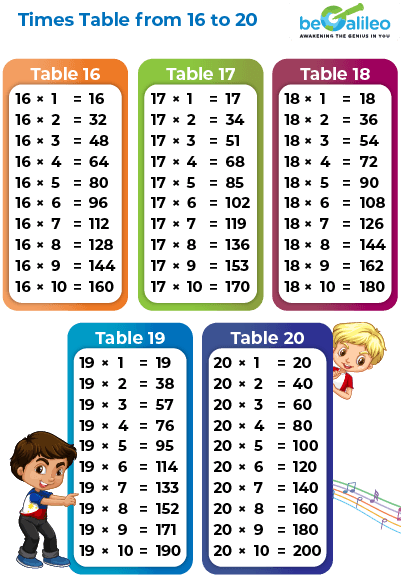## What is a Multiplication chart?

A multiplication chart is a table that displays the product of two numbers. Typically, one set of numbers is written on the vertical column on the left and another set is written along the horizontal row on the top. The products are listed in a rectangular grid, creating an array of numbers.

A multiplication chart of 10 is shown below -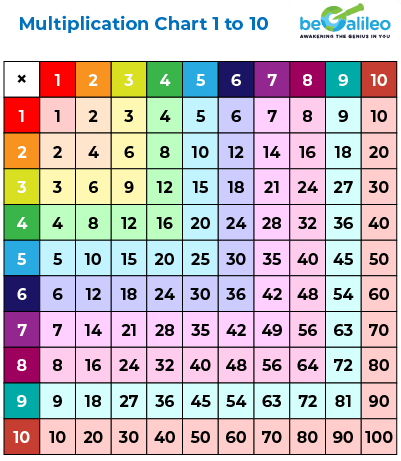### How to read a Multiplication Chart?

Reading a multiplication chart is a valuable skill that helps you quickly find the product of two numbers. Here's a step-by-step guide on how to read a multiplication chart:

Identify the two numbers you want to multiply. One will be along the top row, and the other will be in the left column.
2. Find the Intersection Point :
Trace your finger or eye along the row that contains the first number. Simultaneously, trace your finger or eye down the column that contains the second number.
The point where the row and column intersect will give you the product of the two numbers.
4. Repeat as Needed :
If you have more multiplications to perform, repeat the process for each pair of numbers.
5. Be Mindful of Zero :
If one of the numbers is zero, any product in that row or column will also be zero. It's an important multiplication rule to remember.
6. Practice Mental Math :
As you become more familiar with multiplication, try to use the chart as a reference while gradually developing your mental math skills. This will help you perform calculations more efficiently.

For example, if you want to find the product of 4 and 7 in a multiplication chart, locate 4 along the row and 7 along the column. Trace your fingers or eyes until they meet at the intersection point, and you'll find that the product is 28.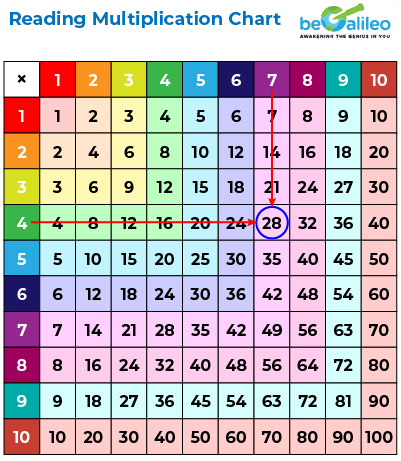Using a multiplication chart can be a helpful tool for learning multiplication facts and performing quick calculations. With practice, you'll become more efficient at reading these charts and eventually transition to mental multiplication for many common calculations.

## Multiplication Times Table Chart ( Free Printable )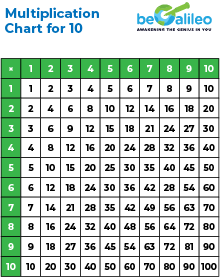## Why is learning multiplication and times tables important? Learning multiplication tables has many benefits. Some of the key benefits of knowing multiplication tables include:

• Foundation for Math Skills : Mastering multiplication tables provides a solid foundation for understanding and performing more complex mathematical operations.
• Quick Mental Calculations : It enables quick mental calculations, making everyday math tasks, like budgeting and shopping, more efficient.
• Boosts Confidence : Knowing times tables instills a sense of achievement, boosting children's self-esteem and confidence in their math abilities.
• Enhances Problem-Solving : It improves problem-solving abilities by breaking down complex problems into manageable steps.
• Preparation for Advanced Math : Multiplication tables serve as a fundamental building block for higher-level math concepts like long division, fractions, and algebra.
• Real-Life Applications : Times tables are used in everyday life, from calculating discounts and recipe measurements to planning events, making them practical and essential skills.

## Easy tricks and tips to learn multiplication tables

Learning multiplication tables can be made easier with these tricks and tips:

• Skip-Counting : Practice skip-counting by saying the multiples out loud. For example, when learning the 7 times table, say 7, 14, 21, 28, and so on. This helps to memorize the sequence.
• Mnemonics : Create mnemonic phrases or stories to remember tricky multiplication facts. For instance, "8 times 7 is 56" can be remembered as "5, 6, pick up sticks."
• Visual Aids : Use multiplication times table charts to visualize multiplication.
• Practice with Flashcards : Use flashcards with multiplication facts on one side and answers on the other side as a fun way to learn multiplication tables.
• Practice Regularly : Consistent practice is key. Set aside a short daily practice session to reinforce the times tables.
• Multiplication Games : Play multiplication games and quizzes online or with friends and family to make learning interactive and engaging.
• Use Patterns : Notice patterns in the multiplication tables. Each multiplication table has its own pattern and knowing that can help memorize the tables faster. For instance, every odd number times an odd number is an odd number.

## Times Tables 1 to 20 ( Free Printables )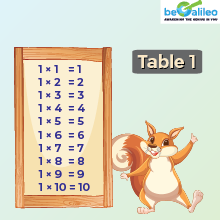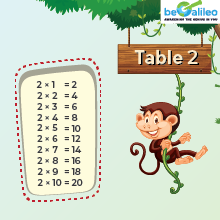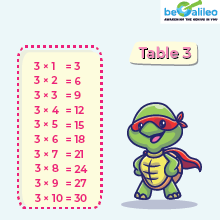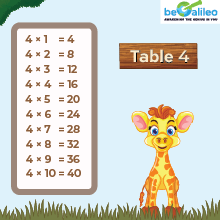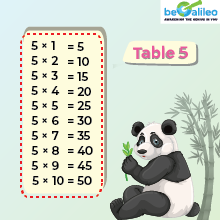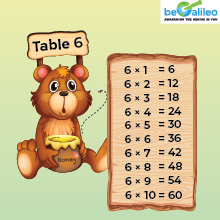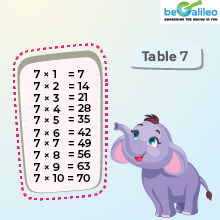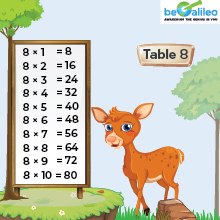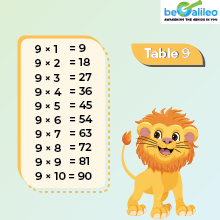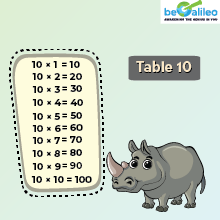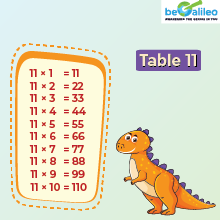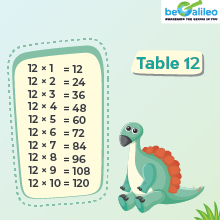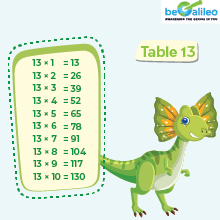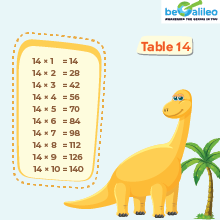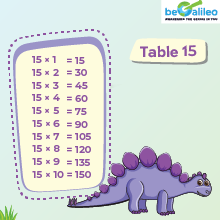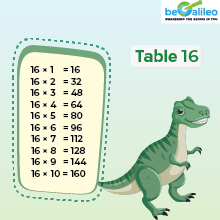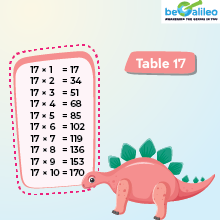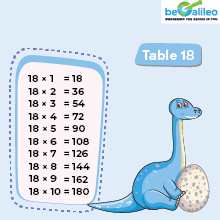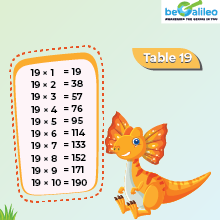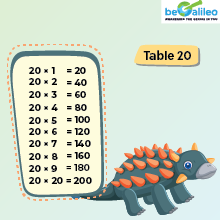## Solved Examples

### Q1. If 6 multiplied by a number equals 24, what is the missing number?

1. a) 6
2. b) 18
3. c) 4
4. d) 12

Solution: The correct answer is c) 4
Let's call the missing number "a." So, 6\times a = 24.
We know that 6\times 4 = 24. Hence the missing number is 4.

### Q2. Find the product of 2 and 10.

1. a) 2 \times 10 = 20
2. b) 2 + 10 = 12
3. c) 10 - 2 = 8
4. d) 2 \times 5 = 10

Solution: The correct answer is a) 2 \times 10 = 20
Look at the multiplication table of 2, and find the product.
Hence, 2 \times 10 = 20 .

### Q3. Sam says the multiplication chart shows the product of 4 \times 5 \text { and } 5 \times 4 are the same. Is he correct?.

1. a) Yes
2. b) No

Solution: The correct answer is a) Yes
Look at the multiplication table of 4 and 5 to find the product of4 \times 5 and 5 \times 4.
Both are 20.
Hence, Sam is correct, as the product of4 \times 5 is the same as the product of5 \times 4

### Q4. Choose the multiplication facts that show the product as 24.

1. a) 3 \times 4
2. b) 9 \times 3
3. c) 12 \times 12
4. d) 6 \times 4

Solution: The correct answer is d) 6 \times 4
Look at the multiplication chart to find the products.
3 \times 4=12 \text{ , } 9 \times 3=27 \text{ , } 12\times12=144\text{ , }and 6\times 4=24
Hence, the multiplication fact that shows the product as 24 is 6\times 4.

### Q5. Sarah wants to buy 3 packs of chocolates, and each pack contains 12 chocolates. How many chocolates will she have in total?

1. a) 24
2. b) 34
3. c) 36
4. d) 15

Solution: The correct answer is c) 36
3 packs\times 12 chocolates/pack= 3 \times12.
Using the multiplication table, we can say 3 \times 12 =36.
Hence Sarah has 36 chocolates in total.

## FAQs - Times Tables

Multiplication times tables are systematic grids that display the results of multiplying each number from 1 to a specific value (usually up to 10, 12, or 20) by another number within that range. They provide a quick reference for multiplication facts.

A multiplication chart is useful because it simplifies the process of finding multiplication answers, making math calculations faster and more efficient. It serves as a handy tool for students, teachers, and anyone who needs to perform multiplication regularly.

Memorizing multiplication tables is crucial because it forms the basis for more advanced math skills. It enables quick mental calculations, enhances problem-solving abilities, and boosts confidence in calculation. It's a fundamental skill for both academic and practical purposes.

To help your child memorize times tables, use strategies like skip-counting, mnemonic devices, flashcards, multiplication songs, visual aids, and regular practice. Make learning fun and engaging to maintain their interest and motivation.

Learning times tables from 1 to 20 may seem daunting, but the key is to break it down into smaller, manageable sections. Focus on one table at a time, practice regularly, and use mnemonic aids or visualizations to aid memorization.

Multiplication tables are used for a wide range of practical applications, including solving mathematical problems, calculating measurements, determining quantities, and budgeting. They are essential in various real-life scenarios that involve multiplication.

Multiplication times tables are important because they provide a strong foundation for mathematical skills. They enhance mental math abilities, facilitate problem-solving, and are applicable in everyday life. Mastery of times tables is a valuable skill that simplifies various mathematical tasks.